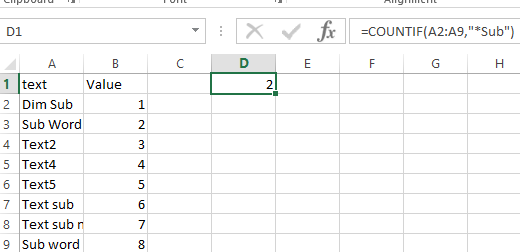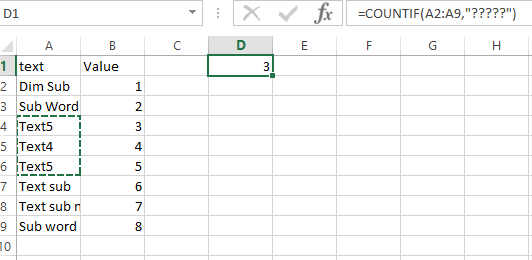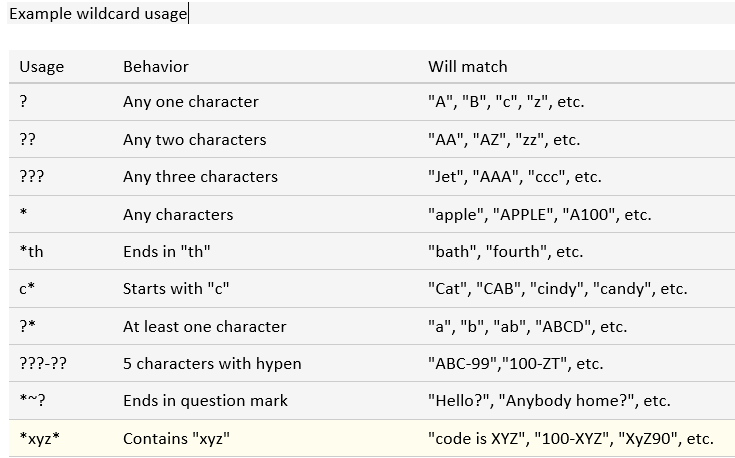#### How to insert a wildcard?

In this article we will learn to insert a wildcard in Excel.

## What is a Wildcard?

A wildcard is a special character that let’s you perform “fuzzy” matching on text in your Excel formulas.

For example, this countif wildcard formula: =COUNTIF(A2:A9,”*sub”)

Let start by creating data for the same.

## How to insert?

Now suppose we want to count the texts in column A which contains the word “sub”, this can be easily done by using wildcard “*sub” in the countif formula: =COUNTIF(A2:A9,”*Sub”)Lets us discuss another common wildcard: =COUNTIF(A2:A9,”?????”)

This will count the cells with 5 alphabet strings:

### Input values:

text

Dim Sub

Sub Word

Text5

Text4

Text5

Text

sub

Text

sub

new Sub

word

Results: 3

Reason:

Text5

Text4

Text5Please find below some of the wildcard examples we generally use:You can use these wildcard characters in formulas such as “IF”, “SEARCH”, “MATCH”, “INDEX”, “VLOOKUP”, etc.

For example, if you want to search for cells that contain “foobar” anywhere in the text, you can use the following formula:

=IF(ISNUMBER(SEARCH(“*foobar*”,A1)), “Yes”, “No”)

Here, the asterisks are acting as wildcard characters and the formula will return “Yes” if the text “excel” is found anywhere in cell A1, and “No” if it’s not.